# 基于FPGA的无线传能数字控制系统的研究Research on Wireless Power Transfer Digital Control System Based on FPGA

DOI: 10.12677/JEE.2019.73019, PDF, HTML, XML, 下载: 325  浏览: 503

Abstract: This paper analyzes the advantages and disadvantages of current wireless energy transfer control systems. In order to solve the detuning problem of the magnetic coupling wireless power transfer system, the working principle of the system is theoretically analyzed, and a frequency tracking control method based on variable mode digital phase locked loop is proposed. The influence of the loop filter on the system response speed and steady-state error is analyzed by Simulink simulation, and the phase-locked speed and phase-locking accuracy of the phase-locked loop are improved by adding the error quantization link in the phase-locked loop. Finally, based on FPGA, the all-digital WPT control system is designed to realize the automatic tracking of the resonant frequency of WPT system, which increases the over-current and over-temperature protection functions of the system. Through simulation and prototype production, it is verified that the system has fast tracking speed and stability, the state error is small, safe and stable.

1. 引言

2. 磁耦合WPT系统失谐机理与分析

2.1. 确立系统拓扑结构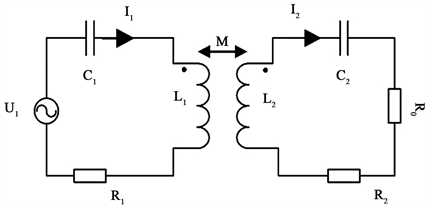Figure 1. WPT equivalent circuit diagram based on coupled mode theory

2.2. 频率跟踪控制原理分析

$\left(\begin{array}{c}{\stackrel{˙}{U}}_{1}\\ 0\end{array}\right)=\left(\begin{array}{cc}{Z}_{1}& -\text{j}\omega M\\ -\text{j}\omega M& {Z}_{2}\end{array}\right)\left(\begin{array}{c}{\stackrel{˙}{I}}_{1}\\ {\stackrel{˙}{I}}_{2}\end{array}\right)$ (1)

${\stackrel{˙}{I}}_{1}=\frac{{\stackrel{˙}{U}}_{1}}{{Z}_{1}+\frac{{\left(2\pi f\right)}^{2}{M}^{2}}{{Z}_{2}}}$ (2)

${\stackrel{˙}{I}}_{2}=\frac{\text{j2}\pi fM{\stackrel{˙}{I}}_{1}}{{Z}_{2}}$ (3)

$\eta =\frac{{\stackrel{˙}{I}}_{2}^{2}{R}_{0}}{{\stackrel{˙}{U}}_{1}{\stackrel{˙}{I}}_{1}}=\frac{{\left(2\pi f\right)}^{2}M{R}_{0}}{\left[{Z}_{1}{Z}_{2}+{\left(2\pi f\right)}^{2}{M}^{2}\right]{Z}_{2}}$ (4)

 (5)

3. 频率跟踪控制系统的设计

3.1. 全数字可变模锁相环的分析与设计

3.1.1. 锁相环的分析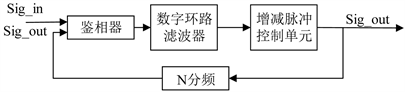Figure 2. Traditional phase-locked loop structure

$H\left(s\right)=\frac{{U}_{o}\left(s\right)}{{U}_{i}\left(s\right)}=\frac{\frac{2{f}_{0}}{kN}}{s+\frac{2{f}_{0}}{kN}}$ (6)

$T=\frac{kN}{2{f}_{0}}$ (7)

$H\left(s\right)=\frac{1}{Ts+1}$ (8)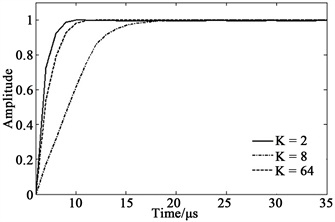Figure 3. Phase-locked loop system step response diagramTable 1. Correspondence between k value and T value

3.1.2. 全数字锁相环的设计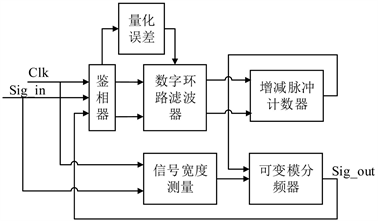Figure 4. Variable-mode all-digital phase-locked loop structure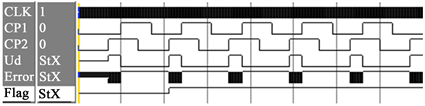(a) 输入信号相位滞后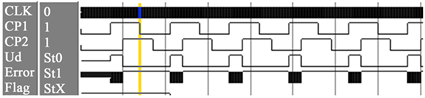(b) 输入信号相位超前

Figure 5. Phase detector simulation

3.2. WPT控制系统的设计与实现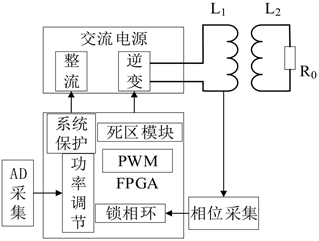Figure 6. Frequency tracking control system structure diagram

4. 控制系统的实现与验证

4.1. 仿真验证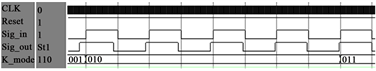Figure 7. Phase-locked loop accuracy simulation diagram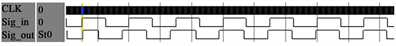(a) 频率由100 Khz变为80 Khz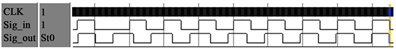(b) 相位改变180度

Figure 8. Phase-locked loop simulation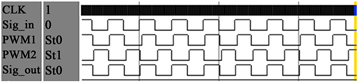Figure 9. Frequency tracking control system simulation diagram

4.2. 系统实验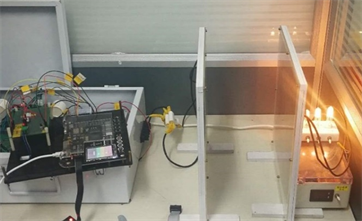Figure 10. Prototype physical mapTable 2. Detailed parameters of the prototype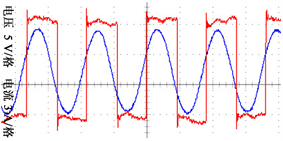(a) 距离为20 cm工作频率为80.4 Khz系统采样图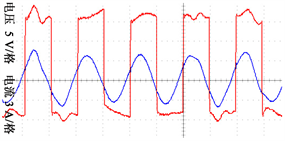(b) 距离为15 cm工作频率为81.8 Khz系统采样图

Figure 11. Transmitting coil voltage and current waveform

5. 结论

  杨庆新, 陈海燕, 徐桂芝, 孙民贵, 傅为农. 无接触电能传输技术的研究进展[J]. 电工技术学报, 2010, 25(7): 6-13.  张波, 疏许健, 黄润鸿. 感应和谐振无线电能传输技术的发展[J]. 电工技术学报, 2017, 32(18): 1-17.  Yin, X., Wang, X. and He, P. (2018) Simulation Analysis and Research of Magnetic Coupling Resonant Wireless Power Transmission System. International Conference on Intelligent Transportation. 2018 International Conference on Intelligent Transportation, Big Data & Smart City, Xiamen, 25-26 January 2018, 772-775. https://doi.org/10.1109/ICITBS.2018.00199  黄学良, 王维, 谭林林. 磁耦合谐振式无线电能传输技术研究动态与应用展望[J]. 电力系统自动化, 2017, 41(2): 2-14+141.  元士强, 崔玉龙, 王景芹, 范好亮, 樊亚超. 磁耦合谐振式无线电能传输系统的阻抗匹配方法研究[J]. 工矿自动化, 2019, 45(1): 81-86.  程泽, 吕月铭, 刘琦, 邢宇涵. 基于磁放大器的耦合谐振式无线电能传输自适应调谐研究[J]. 电工技术学报, 2018, 33(S2): 305-312.  李阳, 董维豪, 杨庆新, 张诚, 刘柳. 过耦合无线电能传输功率降低机理与提高方法[J]. 电工技术学报, 2018, 33(14): 3177-3184.  Stone, D.A., Zhao, R. and Gladwin, D.T. (2016) Maximum Efficiency Point Tracking for Resonant Wireless Power Transfer. IET International Conference on Power Electronics, Glasgow, 19-21 April 2016.  Lim, Y., Tang, H., Lim, S. and Park, J. (2014) An Adaptive Impedance-Matching Network Based on a Novel Capacitor Matrix for Wireless Power Transfer. IEEE Transactions on Power Electronics, 29, 4403-4413. https://doi.org/10.1109/TPEL.2013.2292596  程丽敏, 崔玉龙, 闫贯博. 磁耦合谐振式无线电能传输频率跟踪控制研究[J]. 电力电子技术, 2014, 48(11): 3-6.  傅文珍, 张波, 丘东元. 频率跟踪式谐振耦合电能无线传输系统研究[J]. 变频器世界, 2009(8): 41-46+103.  周豪, 姚钢, 马立伟, 魏文. ICPT系统谐振频率跟踪方法研究[J]. 电力电子技术, 2013, 47(9): 77-79.  刘帼巾, 李义鑫, 崔玉龙, 黄凯, 边鑫磊. 基于FPGA的磁耦合谐振式无线电能传输频率跟踪控制[J]. 电工技术学报, 2018, 33(14): 3185-3193.  单长虹, 孟宪元. 嵌入式自动变模控制的快速全数字锁相环[J]. 计算机仿真, 2004, 21(2): 82-84.